## Each container has a base of 4 1/4 yds by 1 2/3 yds. What is the area covered by all the containers?

Question

Each container has a base of 4 1/4 yds by 1 2/3 yds. What is the area covered by all the containers?

in progress 0
5 months 2021-08-25T18:36:09+00:00 1 Answers 0 views 0

## Answers ( )

See Explanation

Step-by-step explanation:

Given

Base Dimension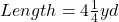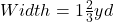Required

The base area of all containers

First, calculate the base area of 1 container.

This is calculated as: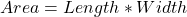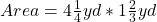Express as improper fraction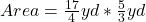So, we have: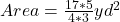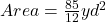The number of containers is not given. So, I will use ‘n’ as the number of containers.

So, we have: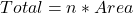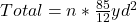——————————————————————————————–

Assume n is 3 (i.e. 3 containers)

The total area is: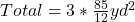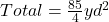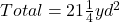——————————————————————————————–